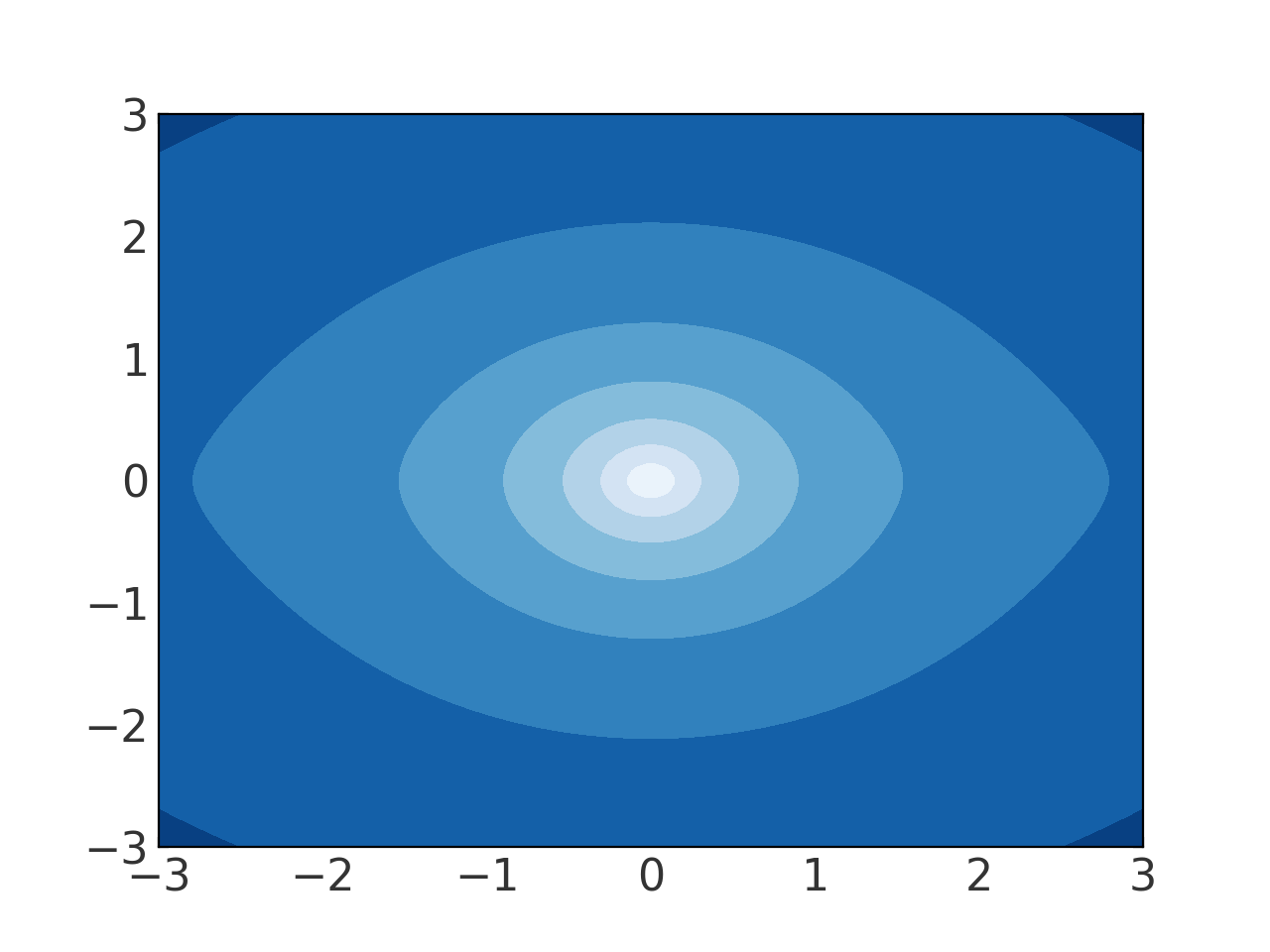# Creating a composite (multi-component ) potential¶

Potential objects can be combined into more complex composite potentials using the CompositePotential or CCompositePotential classes. These classes operate like a Python dictionary in that each component potential must be named, and the potentials can either be passed in to the initializer or added after the composite potential container is already created.

For composing any of the built-in potentials or any external potentials implemented in C, it is always faster to use CCompositePotential, where the composition is done at the C layer rather than in Python.

With either class, interaction with the class is identical. To compose potentials with unique but arbitrary names, you can simply add pre-defined potential class instances:

>>> import numpy as np
>>> import gala.potential as gp
>>> from gala.units import galactic
>>> disk = gp.MiyamotoNagaiPotential(m=1E11, a=6.5, b=0.27, units=galactic)
>>> bulge = gp.HernquistPotential(m=3E10, c=0.7, units=galactic)
>>> pot = disk + bulge
>>> print(pot.__class__.__name__)
CCompositePotential
>>> list(pot.keys())
['c655f07d-a1fe-4905-bdb2-e8a202d15c81',


The two components are assigned unique names and composed into a CCompositePotential instance because the two component potentials are implemented in C (i.e. are CompositePotential instead.

Alternatively, the potentials can be composed directly into the object by treating it like a dictionary. This allows you to specify the keys or names of the components in the resulting CCompositePotential instance:

>>> disk = gp.MiyamotoNagaiPotential(m=1E11, a=6.5, b=0.27, units=galactic)
>>> bulge = gp.HernquistPotential(m=3E10, c=0.7, units=galactic)
>>> pot = gp.CCompositePotential(disk=disk, bulge=bulge)
>>> list(pot.keys())
['disk', 'bulge']


is equivalent to:

>>> pot = gp.CCompositePotential()
>>> pot['disk'] = disk
>>> pot['bulge'] = bulge


In detail, the composite potential classes subclass OrderedDict, so in this sense there is a slight difference between the two examples above (if you are using a Python version < 3.6). By defining components after creating the instance, the order is preserved. In the above example, the disk potential would always be called first and the bulge would always be called second.

The resulting potential object has all of the same properties as individual potential objects:

>>> pot.energy([1.,-1.,0.])
<Quantity [-0.12887588] kpc2 / Myr2>
>>> pot.acceleration([1.,-1.,0.])
<Quantity [[-0.02270876],
[ 0.02270876],
[-0.        ]] kpc / Myr2>
>>> grid = np.linspace(-3.,3.,100)
>>> fig = pot.plot_contours(grid=(grid,0,grid))